The premise of a factor approach to indexes is to construct a stock index that has an intentional and greater exposure to a factor of interest than a given benchmark. By factor, we mean a stock level characteristic such as volatility or value represented by for example, Book to Price. When the benchmark is the Market Portfolio and a positive excess return (or factor premium) exists over the long term, this is often termed a “factor anomaly”, contradicting as it does the “Efficient Market Hypothesis”. Anomalies such as the “value effect” (, ), “size effect” (, ) and “momentum effect” (, ) are well documented. FTSE’s aim is not to justify the existence of such anomalies, but to set out a systematic methodology for the construction of transparent indexes that exhibit an intentional and controlled exposure to the factor(s) of choice.

Interest in a factor approach has been rekindled. This stems from a belief that additional within asset class risk premia exist, and may be captured in a systematic low cost manner [8, 9]. Factor tilts represent a significant component of the value added by traditional active managers and factor approaches to diversification may be more effective in achieving diversification objectives  .

Alternatively weighted indexes frequently exhibit factor tilts. However, typically the objective of such indexes is not factor related. Diversification is the key to avoiding concentration and is the premise on which many alternatively weighted indexes, from Equal Risk Contribution to Maximum Diversification indexes are constructed. Minimum Variance indexes explicitly target reductions in index volatility. Whilst the objective of individual alternatively weighted indexes differs, a common thread is the presence of a specific, non-factor objective.

The historical performance of alternatively weighted indexes has generated huge debate. The consensus is that observed historical out-performance is driven largely by value and small capitalisation factor tilts along with rebalancing gains [5, 6]. This has resulted in a re-evaluation of the merits of factor based approaches to investing and has led some to conclude that alternatively weighted indexes are the appropriate mechanism for accessing factor risk premia.

This is overly simplistic; the majority of alternatively weighted indexes have very specific non-factor objectives; factor outcomes are incidental rather than the result of conscious design choices. The FTSE Minimum Variance indexes typically exhibit small capitalisation and low volatility tilts. The degree to which such tilts are systematic is debatable, but what is undeniable is that the index does not directly target either of these outcomes and the additional complexity of a minimum variance approach is unnecessary for the capture of any low volatility factor premium.

Style and factor indexes have been available for many years, but the role of these original products was largely to fulfill a benchmarking or performance and risk attribution function as opposed to representing replicable indexes, designed to capture the performance of specific factor objectives. FTSE believes that second generation factor indexes should employ a common construction methodology, that directly targets a specific factor objective, with due consideration for diversification, capacity and ease of replication.

Consequently, we emphasise that factor indexes and alternatively weighted indexes are not necessarily interchangeable. A factor index sets out to capture factor exposures in a controlled and considered way. An alternatively weighted index sets out to achieve some other goal and may incidentally achieve factor exposures. For example, a low volatility factor index with exposure to low volatility stocks is not the same as a minimum volatility index. This is clearly seen by noting that the pattern of cross-correlations may allow potentially volatile stocks to represent large weightings in a minimum volatility index, whereas such stocks must necessarily represent a relatively small proportion of any low volatility factor index. The factor index provides exposure to low volatility stocks, whereas the minimum volatility index minimises total index volatility which may (or may not) give incidental exposure to low volatility stocks.

Maximising exposure to a particular factor is not the only criterion of interest; factor indexes must also be “investable”. That is, the degree of capacity, liquidity and concentration are also considerations. For example, if the sole objective is “maximum factor exposure” we would simply devise an index consisting of a single stock with the maximum factor value. Such a maximally undiversified index would be anathema to investors. Indeed the design of factor indexes is further complicated by the fact that factor objectives and investable characteristics are often inversely related. In what follows we demonstrate how to attain a balance across both of these features.

Finally, we address the question of how to create multiple-factor indexes; how does one ensure simultaneous exposure to more than one factor? When there is a reasonable positive correlation between factors, simple addition of individual factor index weights may be sufficient. However, when factors are anti-correlated, such an approach breaks down. To address this problem we introduce the notion of multiple-tilting.

The structure of this document is as follows. In Section 1 we discuss factor design. In Section 2 we set out a methodology for the construction of a single factor index by applying a “tilt” to the weights of some other (underlying) index. In Section 3 we discuss alternative approaches to constructing multiple-factor indexes. In Section 4 we consider the role and application of constraints. Section 5 investigates how the material set out in the previous four Sections may be applied to a concrete example. Finally we summarise our results and conclusions in Section 6.

1. Factor Design and Construction

1.1 Factor Definition

An important determinant of the behaviour of any factor index is the definition of the factor itself. For example should a value factor be based on a single valuation ratio or be comprised of a composite of different ones? Should such a composite comprise “equally-weighted” factors or should it be more heavily weighted to “more important” ones?

The answers to these questions are generally factor specific and preferably based on theoretical work supported by empirical results. The bulk of this document is concerned with what happens after a factor has been created; that is how it is turned into an index. We discuss the detailed construction of Value, Residual Momentum and Quality factors used in the FTSE Global Factor Index Series in a set of separate papers. However, there are some general techniques used during the construction of factors that are important to highlight.

1.2 Factor Neutralisation

Sometimes factor A will be highly correlated with another factor B. One may be interested in defining a “pure A factor” that is not confounded by a signal from factor B. There are various techniques that can be used to remove that part of factor A, which is strongly correlated to factor B.

As an example, consider the case where factor B represents the industrial grouping of a stock. More specifically, assume that factor A is correlated with particular industry groupings and it is desirable to remove this dependence such that any index does not reflect this industry bias. A simple approach is to redefine the stock level factor within a particular industry by measuring it relative to the mean value of the factor within that industry. This ensures all industries are equivalent from a factor perspective. Since the factor is now a relative measure, industry biases in any index based on the re-defined factor will be limited. We discuss a possible application of this, which aids the implementation of Industry and Country constraints, in Section 5.

More sophisticated approaches, which identify independent country and industry factor effects and assess the significance of these effects, are possible.

1.3 Composite Factors

There are several approaches to simultaneously achieving tilts towards multiple factors. The distinction between forming an index based on a composite of indexes and a composite factor is discussed in Section 3.

2. Single Factor Index Methodology

2.1 Calculation of Factor Z-Scores

Consider a universe of stocks U and let fί be some factor that takes real values for stocks within some subset F of U. Then we define the Z-Score of the factor for stocks in the usual way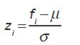where μ and σ are the cross-sectional mean and standard deviation of the factor. Stocks with Z-Scores less than minus three are set to minus three and those with Z-Scores greater than three are set to three. The Z-Scores are then recalculated. This process is repeated iteratively until all remaining stocks have absolute Z-Scores less than or equal to three.

2.2 Mapping Z-Scores to Scores

Z-Scores are now assigned a score, Si Є (0, ) to determine the weights in the factor index. The functional form we use is based on the cumulative normal: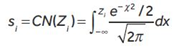If the whole universe is to be weighted, stocks in U – F (i.e. without real factor values) are assigned the score CN (–3) or alternatively assigned a neutral value of CN(0).

The cumulative normal mapping has several advantages over other commonly used mappings, such as: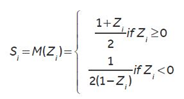An additional mapping that is of interest is: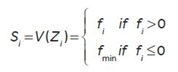for some chosen minimum value fmin.

This mapping can be used to derive a set of “Value Weighted” or “Fundamentally Weighted” indexes, an example of which is discussed in Section 5.

Chart 1 compares mapping schemes based on the cumulative normal CN(Z) and M(Z). The main difference between these two mapping schemes lies in the tails of the Z-Score data. M(Z) tends to overweight stocks with extreme Z-Scores compared to CN(Z). This is undesirable, since extreme Z-Scores are likely to be increasingly unreliable and result in reversals of index weights between index reviews generating unnecessary turnover.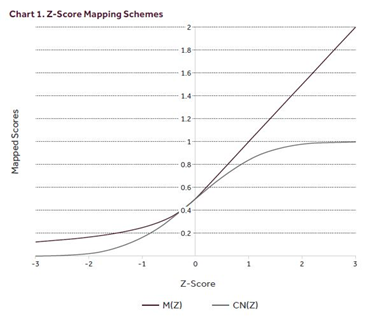2.3 Cumulative Normal Compared to Rank Based Scores

Where a factor is independently and identically normally distributed, the application of the cumulative normal mapping function will yield identical results to the application of a rank based scoring approach in the limit for large sample sizes.

However, where this distributional assumption does not hold and/or for smaller sample sizes, there is a subtle difference between the two approaches; the cumulative normal considers the magnitude of the Z-Score, not just the ranking. This is seen more clearly when one considers that adjacent stocks will have trivially different rankings, whereas the cumulative normal interval between adjacent stocks may be significant.

2.4 Translating Scores to Index Weights

For stocks in the universe U with underlying index weights of Wi, the factor index weights are: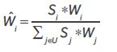The underlying index weights may be of any type; for example they may be Market Capitalisation, Equal or Risk Weights. The resulting factor index can therefore be considered as a “factor overlay” or “factor tilt” on an underlying index. At this stage a minimum weight may be imposed or weights below a minimum threshold set to zero.

To illustrate, consider an equally-weighted underlying index consisting of 1000 stocks and a hypothetical factor whose values have been taken from the normal distribution with mean zero and standard deviation one. Chart 2 shows the resulting distribution of index weights after the application of the CN(Z) and M(Z) mapping schemes. Stocks are ordered left to right from the smallest to largest factor score. Note that M(Z) overweights the tails as expected and shows a potentially problematic concentration in weight for stocks with very large Z-Scores. On the other hand CN(Z) yields a straight line relationship and therefore avoids such concentrated outcomes.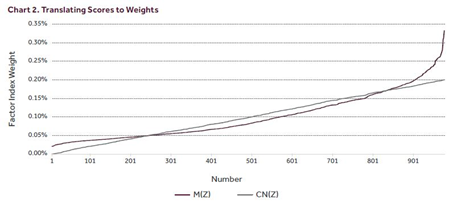The transfer coefficient, representing the efficiency with which the factor signal is reflected in the resulting factor index when M(Z) is used is high at 95%. However, it is higher still when CN(Z) is used, at 98%. In the limit of large sample size the theoretical transfer coefficient values can be shown to be 95.34% and 97.72% ( 3/_ ) respectively.

2.5 Direction of Factor Tilt

A factor index may be tilted in either direction, for example, a long-only low momentum index can be created as opposed to a long-only high momentum index. To create an index that tilts away from a given factor, the sign of a stock’s Z-Score is simply reversed and the score calculated: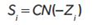This enables the implementation of any view on the prospects of a factor in a long-only context. That is, one can be short a factor without needing to be short the index.

The cumulative normal approach has the additional property that a tilt away from a factor satisfies a symmetry relationship with a tilt towards the same factor. The condition that CN(Zi ) + CN(–Zi ) = 1 ensures that a linear sum of the stock weights of a factor index tilted towards a factor and one tilted away will yield the underlying index weights. The M(Z) scoring function does not share this property.

2.6 Controlling the Strength of a Factor Tilt and Multi-tilting

There are (at least) two ways that the strength of the factor tilt can be altered. Firstly a cumulative normal mapping that has a different standard deviation parameter may be used. The smaller the standard deviation, the stronger the resulting factor tilt. In the limit of zero standard deviation, the cumulative normal function becomes a step function, with zero for negative Z-Scores and one for positive Z-Scores. This results in indexes that consist only of those stocks in the top half of the universe by factor rank, where weights are in identical proportion to the underlying index.

The second method is to perform a “tilt on a tilt”. First we tilt our underlying index toward the factor in the usual way. The resulting weights are now considered as a “new underlying” and are tilted towards the factor again. In this way we create a stronger tilt towards the factor and may continue the process to yield even stronger tilts. Mathematically this can be seen as “exponentiation of the tilt operator”.

We are not forced to use the same factor at each step in the process and therefore can create indexes that are tilted towards more than one factor. It is trivial to show that the order of tilting makes no difference to index outcomes, since a stock’s score in a tilt-tilt index is equal to the product of the separate factor scores. Furthermore, this can be shown explicitly for a multi-tilted index based on the cumulative normal by noting that the score for a stock is given by: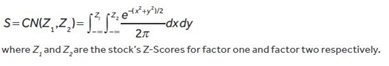2.7 Long/Short Factor Indexes

From an implementation perspective, long-only indexes are preferable to long/short factor indexes. However, long/short factor indexes will provide a stronger tilt towards a factor of interest. A long/short approach can be incorporated within the framework described by combining a long position in a positively tilted index with a short position in the corresponding negatively tilted index. The symmetry property of the Cumulative Normal discussed in Section 2.5 ensures that a short negative factor tilt could at least theoretically be created, by being short the underlying index (future) and long the positive factor index. Chart 3 shows the distribution of long/short weights when this approach is applied to an equally-weighted underlying index with hypothetical factor scores drawn from the normal distribution with mean zero and standard deviation one.